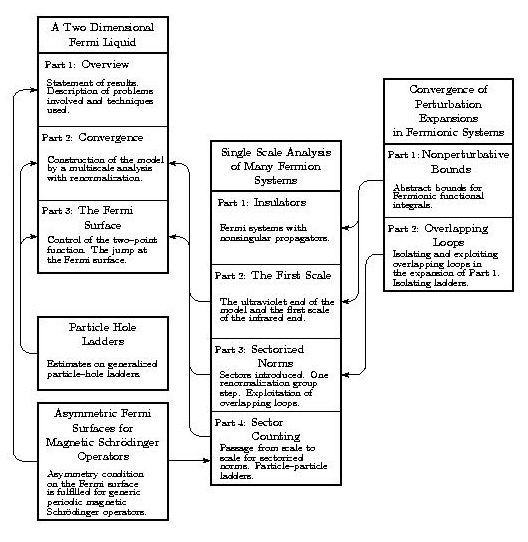Guide to the Fermi Liquid Construction

# Guide to the Fermi Liquid Construction

[ My home page | My research page | Main Fermi liquid construction page ]

This is a short guide to a series of eleven papers in which a construction of an interacting Fermi liquid in two space dimensions is implemented. The main results are stated and proven in [FKTf1, FKTf2, FKTf3]. Theorem I.4 of [FKTf1] gives the convergence of the complete perturbation expansions of the temperature zero connected Green's functions for a system of weakly coupled Fermions with "asymmetric" Fermi surface. An outline of the proof is provided in §2 and § 3 of [FKTf1]. The complete proof - using the results of the auxiliary papers [FKTl], [FKTo1, FKTo2, FKTo3, FKTo4] and [FKTr1, FKTr2] - is given in [FKTf2]. The existence of a discontinuity in the particle number density at the Fermi surface is stated in Theorem I.5 of [FKTf1] and proven in [FKTf3]. Continuity properties of the amputated four point Green's functions, stated in Theorem I.7 of [FKTf1] are also proven in [FKTf3].

Our proof uses a multiscale analysis and discrete renormalization group flow, in the framework of Fermionic functional integrals, and also uses renormalization of the Fermi surface. We introduce a scale parameter M>1 and in the jth step of the renormalization group flow turn on interactions amongst particles whose momenta have distance from the Fermi surface about 1/Mj . The output of the jth step is an effective interaction for particles whose momenta have distance from the Fermi surface less than 1/Mj . In [FKTr1] we build a tool for controlling Gaussian Fermionic functional integrals of exponentials of effective interactions in a quite abstract setting, using Gram bounds for determinants. In [FKTo3, FKTo4] this tool is combined with a technique developed specifically to handle problems created by the fact that the singularity of the original propagator lies on an object with nontrivial geometry, the Fermi surface. The technique is "sectorization of the Fermi surface". It allows us to use the abstract results of [FKTr1] while retaining the ability to exploit conservation of momentum. In particular, conservation of momentum leads to improved power counting, due to "overlapping loops", for the two point function and the non-ladder contributions to the four point function. This mechanism is formulated abstractly in [FKTr2] and implemented concretely in [FKTo3]. Generalized particle-particle and particle-hole ladder diagrams require special treatment. Particle-particle ladders have improved power counting due to the assumed asymmetry of the Fermi surface. This is proven in § XXII of [FKTo4]. The fact that Schrödinger operators with periodic magnetic potentials usually have such asymmetric Fermi surfaces is proven in the auxiliary paper [FKTa]. The estimate on the sum of all contributions from particle-hole ladders exploits a sign cancellation between scales and is developed in [FKTl].

The treatment of the first renormalization group step, i.e. the ultraviolet regime, is a simple application of the expansion of [FKTr1] and appears in [FKTo2]. Control of the change of sectorization forced by the change of scales between renormalization group steps is achieved in [FKTo4].

As mentioned above, [FKTf1] gives an overview of the construction. The paper [FKTo1], where nonsingular propagators (as is the case for insulators) are considered, can also serve as an introduction to some of the techniques. The set of eleven papers is self-contained. [FKTr1+FKTr2], [FKTl] and [FKTo4] are each reasonably independent of the other papers in the series. The interface between each of these and the other papers is through a relatively small number of Definitions and Theorems. The flow chart [pdf,jpg] below gives a thumbnail sketch of the contents of each of the papers and gives the logical connections between the papers.[ My home page | My research page | Main Fermi liquid construction page ]